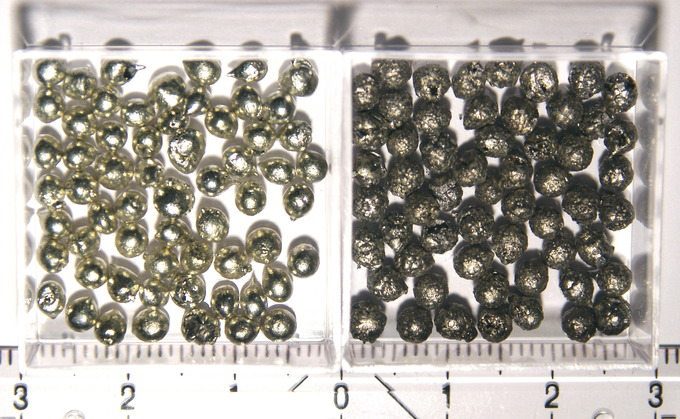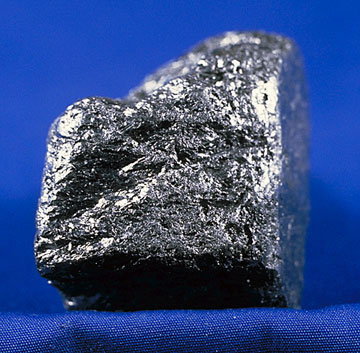## Standard States and Standard Enthalpy Changes

#### Learning Objective

• Calculate the standard enthalpy of formation

#### Key Points

• The standard state of a material is a reference point for the material’s thermodynamic state properties such as enthalpy, entropy, Gibbs free energy, etc. It is used to calculate the material’s properties under different conditions and is denoted as $H^\ominus_f$ .
• The standard state for a gas is the hypothetical state at 1 bar; for liquids and solids, the pure substance at 1 bar; for elements, the most stable allotrope of the element; and for a substance in solution (solute), concentration at 1 M and 1 bar.
• The standard enthalpy of formation is the change in enthalpy that accompanies the formation of one mole of the compound from its elements. The standard enthalpy of reaction occurs in a system when one mole of matter is transformed by a chemical reaction.

#### Terms

• enthalpy of solutionThe heat association with dissolving a particular solute in a particular solvent.
• standard enthalpy of formationThe change in enthalpy that accompanies the formation of one mole of a compound from its elements, with all substances in their standard states; also called “standard heat of formation.”
• standard stateIn chemistry, a reference point used to calculate a material’s (pure substance, mixture, or solution) properties under different conditions.

## Standard States

In chemistry, the standard state of a material, be it a pure substance, mixture, or solution, is a reference point used to calculate its properties under different conditions. In principle, the choice of standard state is arbitrary, although the International Union of Pure and Applied Chemistry (IUPAC) recommends a conventional set of standard states for general use. A standard pressure of 1 bar (101.3 kilopascals) has been accepted.

Strictly speaking, temperature is not part of the definition of a standard state; the standard state of a gas is conventionally chosen to be 1 bar for an ideal gas, regardless of the temperature. However, most tables of thermodynamic quantities are compiled at specific temperatures, most commonly 298.15 K (exactly 25°C) or, somewhat less commonly, 273.15 K (exactly 0°C).

Standard states for atomic elements are given in terms of the most stable allotrope for each element. For example, white tin and graphite are the most stable allotropes of tin and carbon, respectively. Therefore, they are used as standard states or reference points for calculations of different thermodynamic properties of these elements.TinWhite tin (on the left) is the most stable allotrope of tin, and is used as its standard state for thermodynamic calculations.

The standard state should not be confused with standard temperature and pressure (STP) for gases, or with the standard solutions used in analytical chemistry. Standard states are often indicated in textbooks by a circle with a horizontal bar $H^\ominus_f$.GraphiteGraphite is the most stable state of carbon and is used in thermochemistry to define the heat of formation of carbon compounds.

## Standard Enthalpy of Formation

The standard enthalpy of formation, or standard heat of formation, of a compound is the change in enthalpy that accompanies the formation of one mole of the compound from its elements in their standard states. For example, the standard enthalpy of formation for carbon dioxide would be the change in enthalpy for the following reaction:

$C(s)(graphite)+O_2(g)\rightarrow CO_2(g)\quad\quad\quad\quad \Delta H^\ominus_f=-394\; kJ/mol$

Note that standard enthalpies of formation are always given in units of kJ/mol of the compound formed.